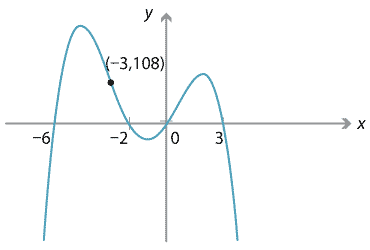## Content

### Polynomials from graphs

Given a polynomial, we have seen how to draw its graph. Now we do the reverse: given a graph, find the polynomial.

It is useful to remember that it takes two points to define a line, and three points to define a parabola. In general, it takes $$n+1$$ points to define a polynomial of degree $$n$$. This is not surprising, since a polynomial of degree $$n$$ has $$n+1$$ coefficients.

#### Example

The graph $$y=f(x)$$ of the quartic polynomial $$f(x)$$ is drawn below. Find $$f(x)$$.Detailed description of diagram

(Reality check: Five points are specified in the graph, namely four $$x$$-intercepts and one extra point. This is the right number of points to determine a quartic polynomial.)

#### Solution

The graph shows four $$x$$-intercepts at $$x=-6,-2,0,3$$. Hence $$(x+6)$$, $$(x+2)$$, $$x$$ and $$(x-3)$$ are all factors of $$f(x)$$. Multiplying these factors together already gives a quartic; the only other possible factor is just a constant. So $$f(x) = c(x+6)(x+2)x(x-3)$$ for some constant $$c$$. Since $$f(-3)=108$$, we have $$108 = c \cdot 3 \cdot (-1) \cdot (-3) \cdot (-6)$$, so $$108 = -54c$$ and $$c=-2$$. Thus $$f(x) = -2(x+6)(x+2)x(x-3)$$.

The above example demonstrates that knowing the roots is not enough information to determine a polynomial. Indeed, in the example, there is a whole family of polynomials $$f(x) = c(x+6)(x+2)x(x-3)$$ with the same roots, where $$c$$ is any (non-zero) real number. We needed the extra data of a point on the curve to determine $$c$$.

In general:

• An $$x$$-intercept at $$x=a$$ implies that $$(x-a)$$ is a factor of $$f(x)$$.
• A $$y$$-intercept at $$y=c$$ implies that $$f(0)=c$$, i.e., the constant term in $$f(x)$$ is $$c$$.
• A given point $$(p,q)$$ on the graph implies that $$f(p)=q$$.

If we are just given points on the graph of $$y=f(x)$$, one way to find the polynomial is by simultaneous equations, as the following example shows.

#### Example

Find the quadratic polynomial $$f(x)$$ whose graph passes through the three points $$(1,-2)$$, $$(2,-1)$$ and $$(3,2)$$.

#### Solution

Let the polynomial be $$f(x) = ax^2 + bx + c$$. We are given that $$f(1)=-2$$, $$f(2)=-1$$ and $$f(3)=2$$, so we have the simultaneous equations

\begin{alignat*}{2} a+b+c &= -2 &\qquad\qquad& (1) \\ 4a+2b+c &= -1 && (2) \\ 9a+3b+c &= 2. && (3) \end{alignat*}

Subtracting $$(2)-(1)$$ and $$(3)-(2)$$ gives $$3a+b = 1$$ and $$5a+b=3$$. Subtracting these two equations gives $$2a=2$$, so $$a=1$$. Substituting $$a=1$$ back into these equations gives $$b=-2$$ and then $$c=-1$$. So $$f(x) = x^2 - 2x - 1$$.

In principle we could use the above method to find, say, a quintic through six given points, or a degree-100 polynomial through 101 given points; it just becomes increasingly tedious to eliminate the variables and solve the simultaneous equations!

As it turns out (and beyond the secondary school curriculum), there is actually a formula for the polynomial through a given set of points, called the Lagrange interpolation formula, discussed in the Appendix section.

Next page - Links forward - Approximating functions